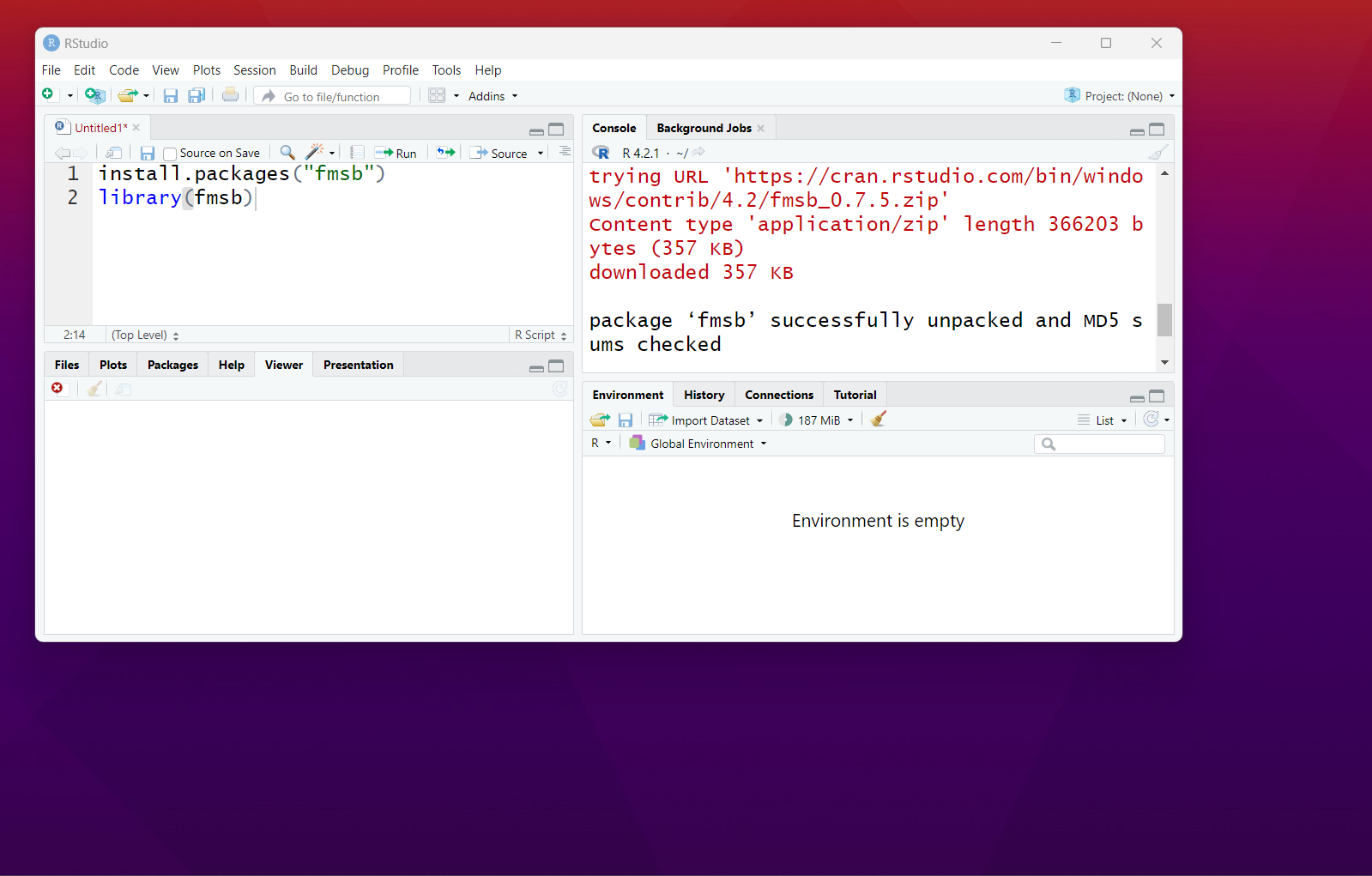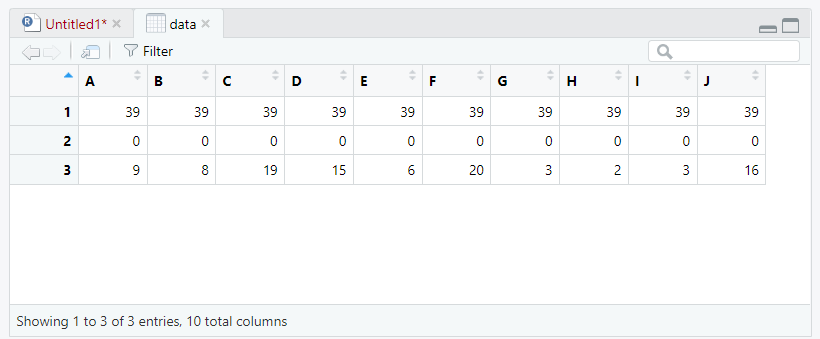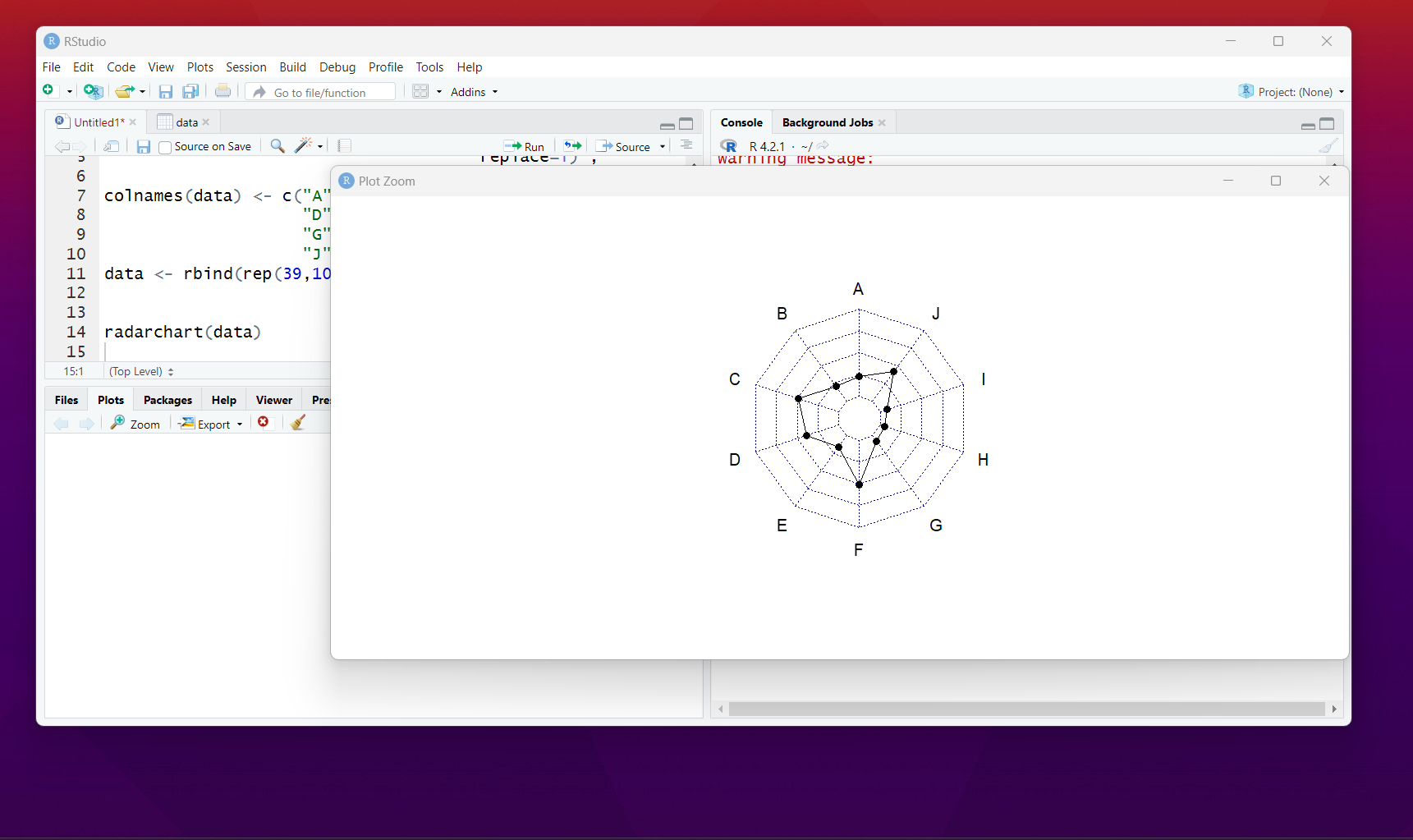# R Language - How to Create Radar chart (spider plot) in R with fmsb

in Education4 months ago

In this post, Learn How to Create Radar chart (spider plot) in R with fmsb. In the previous post, I explained how to use the animated chart with ggplot and plotly. It can be useful for the design of the plots which have timeline like use.

Now let's take a look at the Radar Chart in R. In this Tutorial, we are going to explain how the dataset can be use to make some of the interesting radar plot. For this example, we are making use of the fmsb package which would be used specifially for this purpose.

Let's take a look at the RStudio IDE. It'd be used to draw the plot. Install the package called fmsb. And also we would be making use of the various settings that would help us design and configure the package for the radar plot.

I have created a video to give you an overview on How to Create Radar chart in R with fmsb. You should give this one a try.

First thing we are going to be making use of is the package. So let's try and install the fmsb package for the use of the radar chart.

First thing, is the install of the package.

install.packages("fmsb")
library(fmsb)

This is what we have now.Now we would be in need of the dataset which would help us draw the plot. So check the below dataset.

data <- as.data.frame(matrix( sample( 2:20 , 10 ,
replace=T) ,
ncol=10))
colnames(data) <- c("A" , "B" , "C" ,
"D" , "E", "F" ,
"G" , "H", "I",
"J" )
data <- rbind(rep(39,10) , rep(0,10) , data)

So we have the data ready here.Next thing would be handling the data into our radar chart. And then drawing the plot with the statement.

And we have our first radar chart.That's it. You have the Radar chart in R. You can now check the above video for the rest of the configuration and the settings. Some of the time it is going to be pretty useful for doing various set of the configuration it would be reasonable to try it out.

Next few tutorials would be on more of the libraries like ggplot, plotly and the others. I think once I am done with the R tutorials. I am going to be checking out on the python. So that it would be pretty fun for the data science specific topics.

Another thing is that I need to regularly create the tutorials specific for the anaconda and the R code too. So that most of the content that I make would be specific to the data science and the data visualization. It'd be reasonable that way.

Apart from the data science tutorial. I am also doing the heavy design of the cloud based tutorials. Like say database tutorials and the various cloud cluster deployment. I know in near future thing are going to be fun to watch my videos.

If you happen to like this content, do give me feedback over there and that would help me improve my efforts in near future.Thanks for your contribution to the STEMsocial community. Feel free to join us on discord to get to know the rest of us!

Please consider delegating to the @stemsocial account (85% of the curation rewards are returned).

You may also include @stemsocial as a beneficiary of the rewards of this post to get a stronger support.